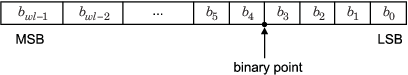## Data Types and Scaling in Digital Hardware

In digital hardware, numbers are stored in binary words. A binary word is a fixed-length sequence of binary digits (1's and 0's). The way in which hardware components or software functions interpret this sequence of 1's and 0's is described by a data type.

Binary numbers are represented as either fixed-point or floating-point data types. A fixed-point data type is characterized by the word size in bits, the binary point, and whether it is signed or unsigned. The position of the binary point is the means by which fixed-point values are scaled and interpreted. With the Fixed-Point Designer™ software, fixed-point data types can be integers, fractionals, or generalized fixed-point numbers. The main difference between these data types is their default binary point. For example, a binary representation of a generalized fixed-point number (either signed or unsigned) is shown below:where

• bi is the ith binary digit.

• wl is the word length in bits.

• bwl-1 is the location of the most significant, or highest, bit (MSB).

• b0 is the location of the least significant, or lowest, bit (LSB).

• The binary point is shown four places to the left of the LSB. In this example, therefore, the number is said to have four fractional bits, or a fraction length of four.

### Binary Point Interpretation

The binary point is the means by which fixed-point numbers are scaled. It is usually the software that determines the binary point. When performing basic math functions such as addition or subtraction, the hardware uses the same logic circuits regardless of the value of the scale factor. In essence, the logic circuits have no knowledge of a scale factor. They are performing signed or unsigned fixed-point binary algebra as if the binary point is to the right of b0.

Fixed-Point Designer supports the general binary point scaling $V=Q*2^E$. `V` is the real-world value, `Q` is the stored integer value, and `E` is equal to `-FractionLength`. In other words, ```RealWorldValue = StoredInteger * 2 ^ -FractionLength```.

`FractionLength` defines the scaling of the stored integer value. The word length limits the values that the stored integer can take, but it does not limit the values `FractionLength` can take. The software does not restrict the value of exponent `E` based on the word length of the stored integer `Q`. Because `E` is equal to `-FractionLength`, restricting the binary point to being contiguous with the fraction is unnecessary; the fraction length can be negative or greater than the word length.

For example, a word consisting of three unsigned bits is usually represented in scientific notation in one of the following ways.

`$\begin{array}{l}bbb.=bbb.×{2}^{0}\\ bb.b=bbb.×{2}^{-1}\\ b.bb=bbb.×{2}^{-2}\\ .bbb=bbb.×{2}^{-3}\end{array}$`

If the exponent were greater than 0 or less than -3, then the representation would involve lots of zeros.

`$\begin{array}{c}bbb00000.=bbb.×{2}^{5}\\ bbb00.=bbb.×{2}^{2}\\ .00bbb=bbb.×{2}^{-5}\\ .00000bbb=bbb.×{2}^{-8}\end{array}$`

These extra zeros never change to ones, however, so they don't show up in the hardware. Furthermore, unlike floating-point exponents, a fixed-point exponent never shows up in the hardware, so fixed-point exponents are not limited by a finite number of bits.

Consider a signed value with a word length of 8, a fraction length of 10, and a stored integer value of 5 (binary value `00000101`). The real-word value is calculated using the formula
`RealWorldValue = StoredInteger * 2 ^ -FractionLength`. In this case, `RealWorldValue = 5 * 2 ^ -10 = 0.0048828125`. Because the fraction length is 2 bits longer than the word length, the binary value of the stored integer is `x.xx00000101` , where `x` is a placeholder for implicit zeros. `0.0000000101` (binary) is equivalent to `0.0048828125` (decimal). For an example using a `fi` object, see Fraction Length Greater Than Word Length.

### Signed Fixed-Point Numbers

Computer hardware typically represents the negation of a binary fixed-point number in three different ways: sign/magnitude, one's complement, and two's complement. Two's complement is the preferred representation of signed fixed-point numbers and is the only representation used by Fixed-Point Designer software.

Negation using two's complement consists of a bit inversion (translation into one's complement) followed by the addition of a one. For example, the two's complement of 000101 is 111011.

Whether a fixed-point value is signed or unsigned is usually not encoded explicitly within the binary word; that is, there is no sign bit. Instead, the sign information is implicitly defined within the computer architecture.

### Floating-Point Data Types

Floating-point data types are characterized by a sign bit, a fraction (or mantissa) field, and an exponent field. Fixed-Point Designer adheres to the IEEE® Standard 754-1985 for Binary Floating-Point Arithmetic (referred to simply as the IEEE Standard 754 throughout this guide) and supports singles and doubles.

When choosing a data type, you must consider these factors:

• The numerical range of the result

• The precision required of the result

• The associated quantization error (i.e., the rounding mode)

• The method for dealing with exceptional arithmetic conditions

These choices depend on your specific application, the computer architecture used, and the cost of development, among others.

With the Fixed-Point Designer software, you can explore the relationship between data types, range, precision, and quantization error in the modeling of dynamic digital systems. With the Simulink® Coder™ product, you can generate production code based on that model. With HDL Coder™, you can generate portable, synthesizable VHDL and Verilog code from Simulink models and Stateflow® charts.

Fixed-point data types can be either signed or unsigned. Signed binary fixed-point numbers are typically represented in one of three ways:

## Related Topics

﻿
##### Support